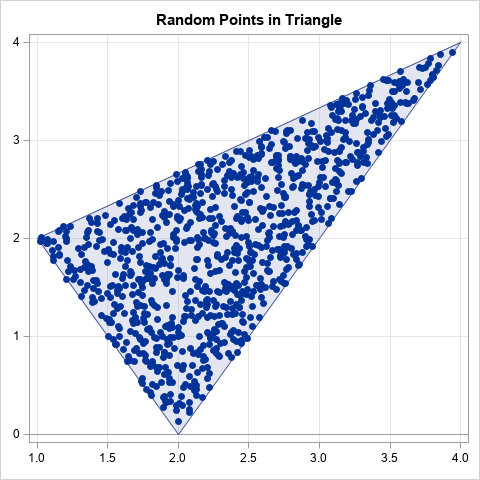How can you efficiently generate N random uniform points in a triangular region of the plane? There is a very cool algorithm (which I call the reflection method) that makes the process easy. I no longer remember where I saw this algorithm, but it is different from the "weighted average" method in Devroye (1986, p. 569-570). This article describes and implements the reflection algorithm for generating random points in a triangle from the uniform distribution. The graph to the right shows 1,000 random points in the triangle with vertices P1=(1, 2), P2=(4, 4), and P3=(2, 0). The method works for any kind of triangle: acute, obtuse, equilateral, and so forth.

In this article, "random points" means that the points are drawn randomly from the uniform distribution.

### Random points in a parallelogram

The easiest way to understand the algorithm is to think about generating points in a parallelogram. For simplicity, translate the parallelogram so that one vertex is at the origin. Two sides of the parallelogram share that vertex. Let a and b be the vectors from the origin to the adjacent vertices.

To produce a random point in the parallelogram, generate u1, u2 ~ U(0,1) and form the vector sum
p = u1*a + u2*b
This is the 2-D parameterization of the parallelogram, so for random u1 and u2, the point p is uniformly distributed in the parallelogram. The geometry is shown in the following figure.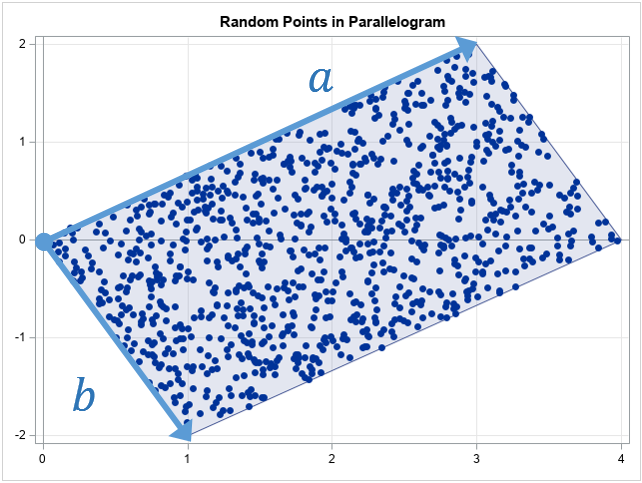The following SAS/IML program generates 1,000 random points in the parallelogram. The graph is shown above.

```proc iml; n = 1000; call randseed(1234);   /* random points in a parallelgram */ a = {3 2}; /* vector along one side */ b = {1 -2}; /* vector along adjacent side */ u = randfun(n // 2, "Uniform"); /* u[,1], u[,2] ~ U(0,1) */ w = u[,1]@a + u[,2]@b; /* linear combination of a and b */ title "Random Points in Parallelogram"; call scatter(w[,1], w[,2]) grid={x,y};```

The only mysterious part of the program is the use of the Kronecker product (the '@' operator) to form linear combinations of the a and b vectors. The details of the Kronecker product operator are described in a separate article. Briefly, it is an efficient way to generate linear combinations of a and b without writing a loop.

### The reflection step

A useful fact about random uniform variates is that if u ~ U(0,1), then also v = 1 - u ~ U(0,1). You can use this fact to convert N points in a parallelogram into N points in a triangle.

Let u1, u2 ~ U(0,1) be random variates in (0,1). If u1 + u2 ≤ 1, then the vector u1*a + u2*b is in the triangle with sides a and b. If u1 + u2 > 1, then define v1 = 1 - u1 and v2 = 1 - u2, which are also random uniform variates. You can verify that the sum v1 + v2 ≤ 1, which means that the vector v1*a + v2*b is in the triangle with sides a and b.

This is shown in the following graph. The blue points are the points for which u1 + u2 ≤ 1. The red points are for u1 + u2 > 1. When you form v1 and v2, the red triangle get reflected twice and ends up on top of the blue triangle. The two reflections are equivalent to a 180 degree rotation about the center of the parallelogram, which might be easier to visualize.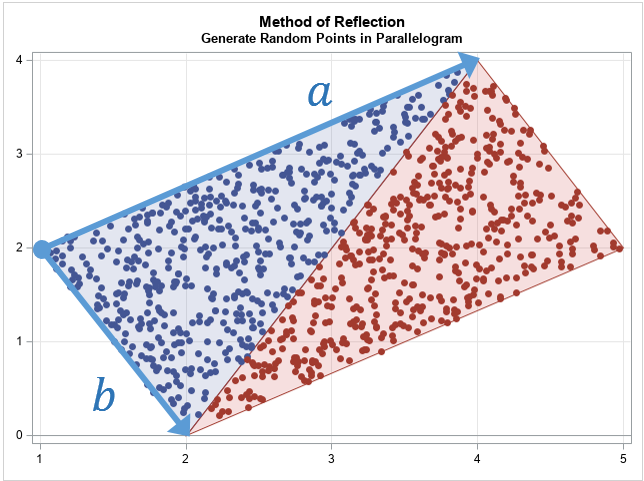### Generate random points in a triangle

With this background, you can now generate random points in any triangle. Let P1, P2, and P3 be the vertices of the triangle. The algorithm to generate random points in the triangle is as follows:

1. Define the vectors a = P2 - P1 and b = P3 - P1. The vectors define the sides of the triangle when it is translated to the origin.
2. Generate random uniform values u1, u2 ~ U(0,1)
3. If u1 + u2 > 1, apply the transformation u1 → 1 - u1 and u2 → 1 - u2.
4. Form w = u1 a + u2 b, which is a random point in the triangle at the origin.
5. The point w + P1 is a random point in the original triangle.

The following SAS/IML program implements this algorithm and runs it for the triangle with vertices P1=(1, 2), P2=(4, 4), and P3=(2, 0).

```/* generate random uniform sample in triangle with vertices P1 = (x0,y0), P2 = (x1,y1), and P3 = (x2,y2) The triangle is specified as a 3x2 matrix, where each row is a vertex. */ start randUnifTriangle(P, n); a = P[2,] - P[1,]; /* translate triangle to origin */ b = P[3,] - P[1,]; /* a and b are vectors at the origin */ u = randfun(n // 2, "Uniform"); idx = loc(u[,+] >= 1); /* identify points outside of the triangle */ if ncol(idx)>0 then u[idx,] = 1 - u[idx,]; /* transform variates into the triangle */ w = u[,1]@a + u[,2]@b; /* linear combination of a and b vectors */ return( P[1,] + w ); /* translate triangle back to original position */ finish; store module=(randUnifTriangle);   /* triangle contains three vertices */ call randseed(1234,1); P = {1 2, /* P1 */ 4 4, /* P2 */ 2 0}; /* P3 */ n = 1000; w = randUnifTriangle(P, n);   title "Random Points in Triangle"; ods graphics / width=480px height=480px; call scatter(w[,1], w[,2]) grid={x,y};```

The graph of the 1,000 random points appears at the top of this program.

### Showing scatter plots with polygons

As written, the programs in this article create scatter plots that show the random points. To improve the exposition, I used the polygon package to draw graphs that overlay the scatter plot and a polygon. You can download and install the polygon package if you have PROC IML with SAS 9.4m3 or later. You can download the complete SAS program that performs all the computations and creates all the graphs in this article.

### Summary

In summary, this article shows how to generate random uniform points in a triangle by using the reflection algorithm. The reflection algorithm is based on generating random points in a parallelogram. If you draw the diagonal of a parallelogram, you get two congruent triangles. The algorithm reflects (twice) all points in one triangle into the other triangle. The algorithm is implemented in SAS by using the SAS/IML language, although you could also use the SAS DATA step.

The post Generate random points in a triangle appeared first on The DO Loop.When my colleague, Robert Allison, blogged about visualizing the Mandelbrot set, I was reminded of a story from the 1980s, which was the height of the fractal craze. A research group in computational mathematics had been awarded a multimillion-dollar grant to purchase a supercomputer. When the supercomputer arrived and got set up, what was the first program they ran? It was a computation of the Mandelbrot set! They wanted to see how fast they could generate pretty pictures with their supercomputer!

Although fractals are no longer in vogue, the desire for fast computations never goes out of style. In a matrix-vector language such as SAS/IML, you can achieve fast computations by vectorizing the computations, which means operating on large quantities of data and using matrix-vector computations. Sometimes this paradigm requires rethinking an algorithm. This article shows how to vectorize the Mandelbrot set computation. The example provides important lessons for statistical computations, and, yes, you get to see a pretty picture at the end!

### The classical algorithm for the Mandelbrot set

As a reminder, the Mandelbrot set is a visualization of a portion of the complex plane. If c is a complex number, you determine the color for c by iterating a complex quadratic map z <- z2 + c, starting with z=0. You keep track of how many iterations it takes before the iteration diverges to infinity, which in practice means that |z| exceeds some radius, such as 5. The parameter values for which the iteration remains bounded belong to the Mandelbrot set. Other points are colored according to the number of iterations before the trajectory exceeded the specified radius.

The classical computation of the Mandelbrot set iterates over the parameter values in a grid, as follows:

```For each value c in a grid: Set z = 0 For i = 1 to MaxIter: Apply the quadratic map, f, to form the i_th iteration, z_i = f^i(z; c). If z_i > radius, stop and remember the number of iterations. End; If the trajectory did not diverge, assume parameter value is in the Mandelbrot set. End;```

An advantage of this algorithm is that it does not require much memory, so it was great for the PCs of the 1980s! For each parameter, the color is determined (and often plotted), and then the parameter is never needed again.

### A vectorized algorithm for the Mandelbrot set

In the classical algorithm, all computations are scalar computations. The outer loop is typically over a large number, K, of grid points. The maximum number of iterations is typically 100 or more. Thus, the algorithm performs 100*K scalar computations in order to obtain the colors for the image. For a large grid that contains K=250,000 parameters, that's 25 million scalar computations for the low-memory algorithm.

A vectorized version of this algorithm inverts the two loops. Instead of looping over the parameters and iterating each associated quadratic map, you can store the parameter values in a grid and iterate all K trajectories at once by applying the quadratic map in a vectorized manner. The vectorized algorithm is:

```Define c to be a large grid of parameters. Initialize a large grid z = 0, which will hold the current state. For i = 1 to MaxIter: For all points that have not yet diverged: Apply the quadratic map, f, to z to update the current state. If any z > radius, assign the number of iterations for those parameters. End; End; If a trajectory did not diverge, assume parameter value is in the Mandelbrot set.```

The vectorized algorithm performs the same computations as the scalar algorithm, but each iteration of the quadratic map operates on a huge number of current states (z). Also, each all states are checked for divergence by using a single call to a vector operation. There are many fewer function calls, which reduces overhead costs, but the vectorized program uses a lot of memory to store all the parameters and states.

Let's see how the algorithm might be implemented in the SAS/IML language. First, define vectorized functions for performing the complex quadratic map and the computation of the complex magnitude (absolute value). Because SAS/IML does not provide built-in support for complex numbers, each complex number is represented by a 2-D vector, where the first column is the real part and the second column is the imaginary part.

```ods graphics / width=720px height=640px NXYBINSMAX=1200000; /* enable large heat maps */ proc iml; /* Complex computations: z and c are (N x 2) matrices that represent complex numbers */ start Magnitude(z); return ( z[,##] ); /* |z| = x##2 + y##2 */ finish;   /* complex iteration of quadratic mapping z -> z^2 + c For complex numbers z = x + iy and c = a + ib, w = z^2 + c is the complex number (x^2 - y^2 + a) + i(2*x*y + b) */ start Map(z, c); w = j(nrow(z), 2, 0); w[,1] = z[,1]#z[,1] - z[,2]#z[,2] + c[,1]; w[,2] = 2*z[,1]#z[,2] + c[,2]; return ( w ); finish;```

The next block of statements defines a grid of parameters for the parameter, c:

```/* Set parameters: initial range for grid of points and number of grid divisions Radius for divergence and max number of iterations */ nRe = 541; /* num pts in Real (horiz) direction */ nIm = 521; /* num pts in Imag (vert) direction */ re_min = -2.1; re_max = 0.6; /* range in Real direction */ im_min = -1.3; im_max = 1.3; /* range in Imag direction */ radius = 5; /* when |z| > radius, iteration has diverged */ MaxIter = 100; /* maximum number of iterations */   d_Re = (Re_max - Re_min) / (nRe-1); /* horiz step size */ d_Im = (Im_max - Im_min) / (nIm-1); /* vert step size */ Re = do(re_min, re_max, d_Re); /* evenly spaced array of horiz values */ Im = do(im_min, im_max, d_Im); /* evenly spaced array of vert values */   c = expandgrid( re, im); /* make into 2D grid */ z = j(nrow(c), 2, 0); /* initialize z = 0 for grid of trajectories */ iters = j(nrow(c), 1, .); /* for each parameter, how many iterations until diverge */```

In this example, the parameters for the mapping are chosen in the rectangle with real part [-2.1, 0.6] and imaginary part [-1.3, 1.3]. The parameter grid contains 541 horizontal values and 521 vertical values, for a total of almost 282,000 parameters.

The vectorized program will iterate all 282,000 mappings by using a single call to the Map function. After each iteration, all trajectories are checked to see which ones have left the disk of radius 5 (diverged). The iters vector stores the number of iterations until leaving the disk. During the iterations, a missing value indicates that the trajectory has not yet left the disk. At the end of the program, all trajectories that have not left the disk are set to the maximum number of iterations.

There are two efficiencies you can implement. First, you can pre-process some of the parameters that are known to be inside the "head" or "body" of the bug-shaped Mandelbrot set. Second, for each iteration, you only need to apply the quadratic map to the points that have not yet diverged (that is, iters is missing for that parameter). This is implemented in the following program statements:

```/* Pre-process parameters that are known to be in Mandelbrot set. Params in "head": inside circle of radius 1/8 centered at (-1, 0) Params in "body": inside rect [-0.5, 0.2] x [-0.5, 0.5] */ idxHead = loc( (c - {-1 0})[,##] < 0.0625 ); if ncol(idxHead) > 0 then iters[idxHead] = MaxIter; idxBody = loc(c[,1]> -0.5 & c[,1]< 0.2 & c[,2]> -0.5 & c[,2]< 0.5); if ncol(idxBody) > 0 then iters[idxBody] = MaxIter;   /* compute the Madelbrot set */ done = 0; /* have all points diverged? */ do i = 1 to MaxIter until(done); j = loc(iters=.); /* find the points that remain bounded */ w = z[j,]; a = c[j,]; /* keep only states and parameters tht have not diverged */ w = Map(w, a); /* map those points forward one iteration */ z[j,] = w; /* update current state */ mag = Magnitude(w); /* compute magnitude of current states */ diverged = (mag >= radius) & (iters[j] = .); /* which points diverged on this iteration? */ idx = loc(diverged); /* indices of the diverged points */ if ncol(idx)> 0 then /* if diverged, remember the iteration number */ iters[j[idx]] = i; if all(diverged > 0) then done = 1; end; /* Points that never diverged are part of the Mandelbrot set. Assign them MaxIter */ idx = loc(iters=.); if ncol(idx)>0 then iters[idx] = MaxIter;```

The last step is to assign colors that visualize the Mandelbrot set. Whereas Robert Allison used a scatter plot, I will use a heat map. The iters vector contains the number of iterations before divergence, or MaxIters if the trajectory never diverged. You can assign a color ramp to the number of iterations, or, as I've done below, to a log-transformation of the iteration number. I've previously discussed several ways to assign colors to a heat map.

```/* Color parameter space by using LOG10(number of iterations) */ numIters = log10( shape(iters, nRe, nIm) ); /* shape iterations into matrix */ mandelbrot = numIters`; /* plot num iterations for each parameter */ call heatmapcont(mandelbrot) xValues=Re yValues=Im ColorRamp="Temperature" displayoutlines=0 showlegend=0 title="Mandelbrot Set from Vector Computations";```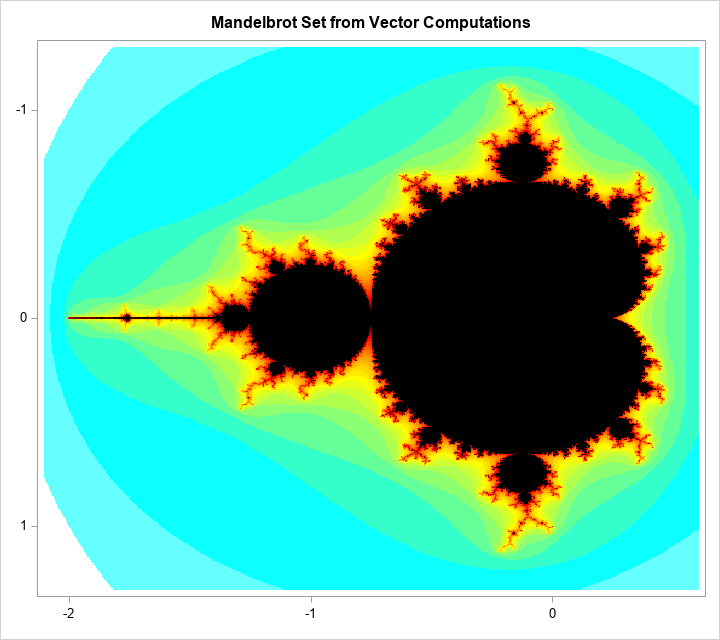On my PC, the Mandelbrot computation takes about a second. This is not blazingly fast, but it is faster than the low-memory, non-vectorized, computation. If you care about the fastest speeds, use the DATA step, as shown in Robert's blog post.

I'm certainly not the first person to compute the Mandelbrot set, so what's the point of this exercise? The main purpose of this article is to show how to vectorize an iterative algorithm that performs the same computation many times for different parameter values. Rather than iterate over the set of parameter values and perform millions of scalar computations, you create an array (or matrix) of parameter values and perform a small number of vectorized computations. In most matrix-vector programming languages, a vectorized computation is more efficient than looping over each parameter value. There are few function calls and each call performs high-level computations on large matrices and vectors.

Statistical programmers don't usually have a need to compute fractals, but the ideas in this program apply to time series computations, root-finding, optimization, and any computation where you compute the same quantity for many parameter values. In short, although the Mandelbrot set is fun to compute, the ability to vectorize computations in a matrix language is a skill that rewards you with more than pretty pictures. And fast computations never go out of style.

The post Vectorize the computation of the Mandelbrot set in a matrix language appeared first on The DO Loop.Modern statistical software provides many options for computing robust statistics. For example, SAS can compute robust univariate statistics by using PROC UNIVARIATE, robust linear regression by using PROC ROBUSTREG, and robust multivariate statistics such as robust principal component analysis. Much of the research on robust regression was conducted in the 1970s, so I was surprised to learn that a robust version of simple (one variable) linear regression was developed way back in 1950! This early robust regression method uses many of the same techniques that are found in today's "modern" robust regression methods. This article describes and implements a robust estimator for simple linear regression that was developed by Theil (1950) and extended by Sen (1968).

### The Theil-Sen robust estimator

I had not heard of the Theil-Sen robust regression method until recently, perhaps because it applies only to one-variable regression. The Wikipedia article about the Theil-Sen estimator states that the method is "the most popular nonparametric technique for estimating a linear trend" in the applied sciences due to its "simplicity in computation, ... robustness to outliers," and "[limited assumptions]regarding measurement errors."

The idea behind the estimator is simple. If the data contains N pairs of (x, y) values, compute all the slopes between pairs of points and choose the median as the estimate of the regression slope. Using that slope, pass a line through each pair of (x,y) values to obtain N intercepts. Choose the median of the intercepts as the estimate of the regression intercept.

That's it. You compute "N choose 2" (which is N*(N-1)/2) slopes and take the median. Then compute N intercepts and take the median. The slope estimate is unbiased and the process is resistant to outliers.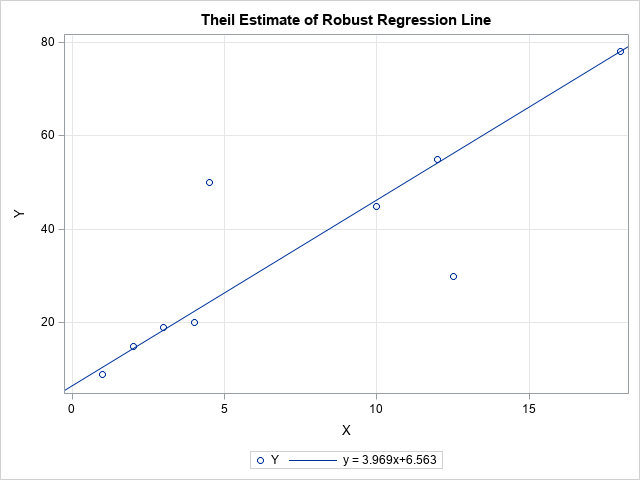The adjacent scatter plot shows the Theil-Sen regression line for nine data points. The seven data points that appear to fall along the regression line were used by Sen (1968). I added two outliers. The plot shows that the Theil-Sen regression line ignores the outliers and passes close to the other data points. The slope of the Theil-Sen line is slightly less than 4. In contrast, the least squares line through these data has a slope of only 2.4 because of the influence of the two outliers.

### Implement the Theil-Sen estimator in SAS

You can easily implement Theil-Sen regression in SAS/IML, as follows:

1. Use the ALLCOMB function to generate all pairs of the values {1, 2, ..., N}. Or, for large N, use the RANDCOMB function to sample pairs of values.
2. Use subscript operations to extract the pairs of points. Compute all slopes between the pairs of points.
3. Use the MEDIAN function to compute the median slope and the median intercept.

The following SAS/IML program implements this algorithm. The program is very compact (six statements) because it is vectorized. There are no explicit loops.

```proc iml; XY = {1 9, 2 15, 3 19, 4 20, 10 45, 12 55, 18 78, /* 7 data points used by Sen (1968) */ 12.5 30, 4.5 50}; /* 2 outliers (not considered by Sen) */   /* Theil uses all "N choose 2" combinations of slopes of segments. Assume that the first coordinates (X) are distinct */ c = allcomb(nrow(XY), 2); /* all "N choose 2" combinations of pairs */ Pt1 = XY[c[,1],]; /* extract first point of line segments */ Pt2 = XY[c[,2],]; /* extract second point of line segments */ slope = (Pt1[,2] - Pt2[,2]) / (Pt1[,1] - Pt2[,1]); /* Careful! Assumes x1 ^= x2 */ m = median(slope); b = median( XY[,2] - m*XY[,1] ); /* median(y-mx) */ print (b||m)[c={'Intercept' 'Slope'} L="Method=Theil Combs=All"];```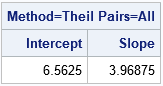As stated earlier, the Theil-Sen estimate has a slope of 3.97. That value is the median of the slopes among the 36 line segments that connect pairs of points. The following graphs display the 36 line segments between pairs of points and a histogram of the distribution of the slopes. The histogram shows that the value 3.97 is the median value of the distribution of slopes.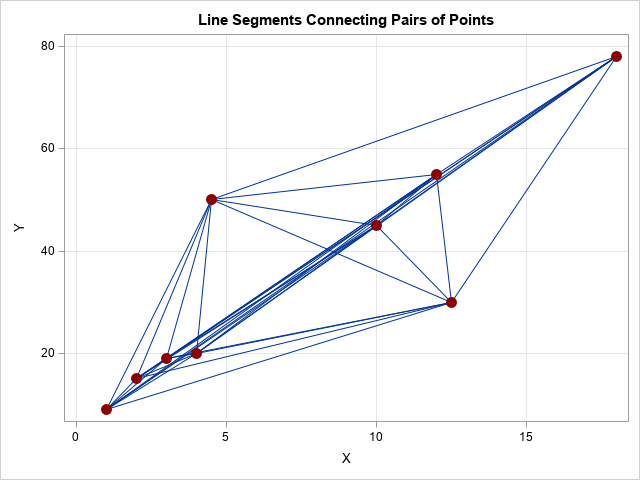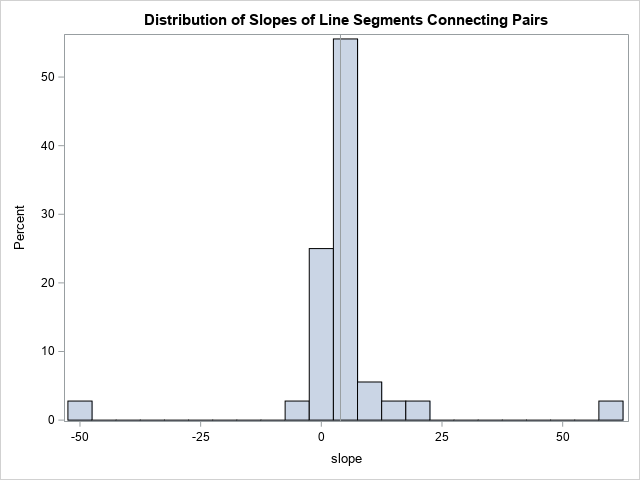### Handling repeated X values: Sen's extension

The observant reader might object that the slopes of the line segments will be undefined if any of the data have identical X values. One way to deal with that situation is to replace the undefined slopes by large positive or negative values, depending on the sign of the difference between the Y values. Since the median is a robust estimator, adding a few high and low values will not affect the computation of the median slope. Alternatively, Sen (1968) proved that you can omit the pairs that have identical X values and still obtain an unbiased estimate. In the following SAS/IML program, I modified the X values of the two outliers so that only seven of the nine X values are unique. The LOC function finds all pairs that have different X values, and only those pairs are used to compute the robust regression estimates.

```/* Sen (1968) handles repeated X coords by using only pairs with distinct X */ XY = {1 9, 2 15, 3 19, 4 20, 10 45, 12 55, 18 78, 12 30, 4 50}; /* last two obs are repeated X values */ c = allcomb(nrow(XY), 2); /* all "N choose 2" combinations of pairs */ Pt1 = XY[c[,1],]; /* first point of line segments */ Pt2 = XY[c[,2],]; /* second point of line segments */ idx = loc(Pt1[,1]-Pt2[,1]^=0); /* find pairs with same X value */ Pt1 = Pt1[idx,]; /* keep only pairs with different X values */ Pt2 = Pt2[idx,];   slope = (Pt1[,2] - Pt2[,2]) / (Pt1[,1] - Pt2[,1]); m = median(slope); b = median( XY[,2] - m*XY[,1] ); /* median(y-mx) */ print (b||m)[c={'Intercept' 'Slope'} L="Method=Sen Combs=All"];```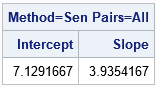### A function to compute the Theil-Sen estimator

The following function defines a SAS/IML function that implements the Theil-Sen regression estimator. I added two options. You can use the METHOD argument to specify how to handle pairs of points that have the same X values. You can use the NUMPAIRS option to specify whether to use the slopes of all pairs of points or whether to use the slopes of K randomly generated pairs of points.

```proc iml; /* Return (intercept, slope) for Theil-Sen robust estimate of a regression line. XY is N x 2 matrix. The other arguments are: METHOD: If method="Theil" and a pair of points have the same X coordinate, assign a large positive value instead of +Infinity and a large negative value instead of -Infinity. If method="Sen", omit any pairs of points that have the same first coordinate. NUMPAIRS: If numPairs="All", generate all "N choose 2" combinations of the N points. If numPairs=K (positive integer), generate K random pairs of points. */ start TheilSenEst(XY, method="SEN", numPairs="ALL"); Infinity = 1e99; /* big value for slope instead of +/- infinity */ if type(numPairs)='N' then c = rancomb(nrow(XY), 2, numPairs); /* random combinations of pairs */ else if upcase(numPairs)="ALL" then c = allcomb(nrow(XY), 2); /* all "N choose 2" combinations of pairs */ else stop "ERROR: The numPairs option must be 'ALL' or a postive integer";   Pt1 = XY[c[,1],]; /* first points for slopes */ Pt2 = XY[c[,2],]; /* second points for slopes */ dy = Pt1[,2] - Pt2[,2]; /* change in Y */ dx = Pt1[,1] - Pt2[,1]; /* change in X */ idx = loc( dx ^= 0 ); if upcase(method) = "SEN" then do; /* exclude pairs with same X value */ slope = dy[idx] / dx[idx]; /* slopes of line segments */ end; else do; /* assign big slopes for pairs with same X value */ slope = j(nrow(Pt1), 1, .); /* if slope calculation is 0/0, assign missing */ /* Case 1: x1 ^= x2. Do the usual slope computation */ slope[idx] = dy[idx] / dx[idx]; /* Case 2: x1 = x2. Assign +Infinity if sign(y1-y2) > 0, else assign -Infinity */ jdx = loc( dx = 0 & sign(dy)>0 ); if ncol(jdx)>0 then slope[jdx] = Infinity; jdx = loc( dx = 0 & sign(dy)<0 ); if ncol(jdx)>0 then slope[jdx] = -Infinity; end; m = median(slope); b = median( XY[,2] - m*XY[,1] ); /* median(y-mx) */ return( b || m ); finish;   /* Test all four calls */ XY = {1 9, 2 15, 3 19, 4 20, 10 45, 12 55, 18 78, 18 30, 4 50}; /* last two obs are outliers not considered by Sen */   est = TheilSenEst(XY, "Theil", "All"); print est[c={'Intercept' 'Slope'} L="Method=Theil; Pairs=All"];   est = TheilSenEst(XY, "Sen", "All"); print est[c={'Intercept' 'Slope'} L="Method=Sen; Pairs=All"];   call randseed(123, 1); est = TheilSenEst(XY, "Theil", 200); print est[c={'Intercept' 'Slope'} L="Method=Theil; Pairs=200"];   call randseed(123, 1); est = TheilSenEst(XY, "Sen", 200); print est[c={'Intercept' 'Slope'} L="Method=Sen; Pairs=200"]; QUIT;```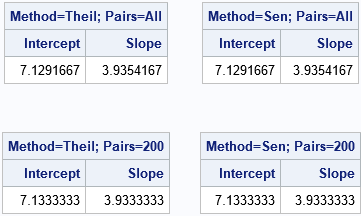For these data, the estimates are the same whether you exclude pairs of points that have identical X coordinates or whether you replace the undefined slopes with large values. For this small data set, there is no reason to use the randomly chosen pairs of points, but that syntax is shown for completeness. Of course, if you run the analysis with a different random number seed, you will get a different estimate.

### Summary

Although Theil published the main ideas for this method in 1950, it contains many of the features of modern robust statistical estimates. Specifically, a theme in modern robust statistics is to exhaustively or randomly choose many small subsets of the data. You compute a (classical) estimate on each subset and then use the many estimates to obtain a robust estimate. I did not realize that Theil had introduced these basic ideas almost seventy years ago!

Theil and Sen also included confidence intervals for the estimates, but I have not included them in this brief article.

### References

The post The Theil-Sen robust estimator for simple linear regression appeared first on The DO Loop.Many statistical tests use a CUSUM statistic as part of the test. It can be confusing when a researcher refers to "the CUSUM test" without providing details about exactly which CUSUM test is being used. This article describes a CUSUM test for the randomness of a binary sequence. You start with a long sequence of binary values such as heads and tails from a coin toss. The test tries to determine whether the sample comes from a Bernoulli distribution with probability p=0.5. In short, is the binary sequence random?

The CUSUM test for randomness of a binary sequence is one of the NIST tests for verifying that a random or pseudorandom generator is generating bits that are indistinguishable from truly random bits (Rukin, et al., 2000, revised 2010, pp 2-31 through 2-33). The test is straightforward to implement. You first translate the data to {-1, +1} values. You then compute the cumulative sums of the sequence. If the sequence is random, the cumulative sum is equivalent to the position of a random walker who takes unit steps. If the sequence is random, the sums will not move away from 0 (the expected sum) too quickly. I've previously visualized the random walk with unit steps (sometimes called a "Drunkard's walk").

Before proceeding to the CUSUM test, I should mention that this test is often used in conjunction with other tests, such as the "runs test" for randomness. That is because a perfectly alternating sequence such as 0101010101... will pass the CUSUM test even though the sequence is clearly not randomly generated. In fact, any sequence that repeatedly has k zeros followed by k ones also passes the test, provided that k is small enough.

### The CUSUM test for randomness of a binary sequence in SAS

The NIST report contains an example of calling the CUSUM test with a sequence of length N=100. The following SAS/IML statements define a sequence of {0, 1} values, convert those values to {-1, +1}, and plot the cumulative sums:

```proc iml; eps = {1 1 0 0 1 0 0 1 0 0 0 0 1 1 1 1 1 1 0 1 1 0 1 0 1 0 1 0 0 0 1 0 0 0 1 0 0 0 0 1 0 1 1 0 1 0 0 0 1 1 0 0 0 0 1 0 0 0 1 1 0 1 0 0 1 1 0 0 0 1 0 0 1 1 0 0 0 1 1 0 0 1 1 0 0 0 1 0 1 0 0 0 1 0 1 1 1 0 0 0 }; x = 2*eps - 1; /* convert to {-1, +1} sequence */ S = cusum(x);   title "Cumulative Sums of Sequence of {-1, +1} Values"; call series(1:ncol(S), S) option="markers" other="refline 0 / axis=y" label="Observation Number";```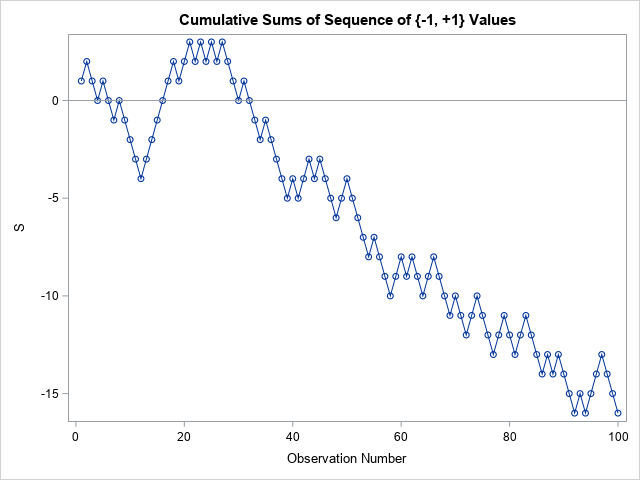The sequence contains 58 values of one category and 42 values of the other. For a binomial distribution with p=0.5, the probability of a sample that has proportions at least this extreme is about 13%, as shown by the computation 2*cdf("Binomial", 42, 0.5, 100);. Consequently, the proportions are not unduly alarming. However, to test whether the sequence is random, you need to consider not only the proportion of values, but also the sequence. The graph of the cumulative sums of the {-1, +1} sequence shows a drift away from the line S=0, but it is not clear from the graph whether the deviation is more extreme than would be expected for a random sequence of this length.

The CUSUM test gives you a way to quantify whether the sequence is likely to have occurred as a random draw from a Bernoulli(p=0.5) distribution. The test statistic is the maximum deviation from 0. As you can see from the graph, the test statistic for this sequence is 16. The NIST paper provides a formula for the probability that a statistic at least this extreme occurs in a random sequence of length N=100. I implemented a (vectorized) version of the formula in SAS/IML.

```/* NIST CUSUM test for randomness in a binary {-1, +1} sequence. https://nvlpubs.nist.gov/nistpubs/legacy/sp/nistspecialpublication800-22r1a.pdf Section 2.13. Pages 2-31 through 2-33. INPUT: x is sequence of {-1, +1} values. */ start BinaryCUSUMTest(x, PrintTable=1, alpha=0.01); S = colvec( cusum(x) ); /* cumulative sums */ n = nrow(S); z = max(abs(S)); /* test statistic = maximum deviation */   /* compute probability of this test statistic for a sequence of this length */ zn = z/sqrt(n); kStart = int( (-n/z +1)/4 ); kEnd = int( ( n/z -1)/4 ); k = kStart:kEnd; sum1 = sum( cdf("Normal", (4*k+1)*zn) - cdf("Normal", (4*k-1)*zn) );   kStart = int( (-n/z -3)/4 ); k = kStart:kEnd; sum2 = sum( cdf("Normal", (4*k+3)*zn) - cdf("Normal", (4*k+1)*zn) ); pValue = 1 - sum1 + sum2;   /* optional: print the test results in a nice human-readable format */ cusumTest = z || pValue; if PrintTable then do; print cusumTest[L="Result of CUSUM Test" c={"Test Statistic" "p Value"}]; labl= "H0: Sequence is a random binary sequence"; if pValue <= alpha then msg = "Reject H0 at alpha = " + char(alpha); /* sequence seems random */ else msg = "Do not reject H0 at alpha = " + char(alpha); /* sequence does not seem random */ print msg[L=labl]; end; return ( cusumTest ); finish;   /* call the function for the {-1, +1} sequence */ cusumTest = BinaryCUSUMTest(x);```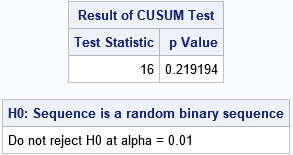According to the CUSUM test, there is not sufficient evidence to doubt that the sequence was generated from a random Bernoulli process.

• if you vectorize the computations, the CUSUM test requires only a few SAS/IML statements. Half of the function is dedicated to printing the results in a friendly format.
• The computation of the p-value took me a while to puzzle over. The formulas in the NIST article did not write the INT() function for the limits of the summation. But the summation only makes sense when the index of summation (k) is an integer.
• The significance value for the CUSUM test is usually chosen to be alpha = 0.01.
• The CUSUM test depends only on the maximum deviation of the cumulative sums (the test statistic) and on the length of the sequence. For a sequence of length 100, the test statistic can be as large as 28 without rejecting the null hypothesis. If the statistic is 29 or larger, then the null hypothesis is rejected and we conclude that the sequence is not generated by a random process.

A neat thing about the CUSUM test is that you can compute the maximum test statistic based only on the sequence length. Thus if you plan to toss a coin 100 times to determine if it is fair, you can stop tossing (with 99% confidence) if the number of heads ever exceeds the number of tails by 29. Similarly, you can stop tossing if you know that the number of excess heads cannot possibly be 29 or greater. (For example, you've tossed 80 times and the current cumulative sum is 5.) You can apply the same argument to excess tails.

In summary, this article shows how to implement the CUSUM test for randomness of a binary sequence in SAS. Only a few lines of SAS/IML are required, and you can implement the test without using any loops. Be aware that the CUSUM test is not very powerful because regular sequences can pass the test. For example, the sequence 000111000111000111... has a maximum deviation of 3.

The post The CUSUM test for randomness of a binary sequence appeared first on The DO Loop.Suppose you need to assign 100 patients equally among 3 treatment groups in a clinical study. Obviously, an equal allocation is impossible because the second number does not evenly divide the first, but you can get close by assigning 34 patients to one group and 33 to the others. Mathematically, this is a grade-school problem in integer division: simply assign floor(100/3) patients to each group, then deal with the remainders. The FLOOR function rounds numbers down.

The problem of allocating a discrete number of items to groups comes up often in computer programming. I call the solution the FLOOR-MOD trick because you can use the FLOOR function to compute the base number in each group and use the MOD function to compute the number of remainders. Although the problem is elementary, this article describes two programming tips that you can use in a vectorized computer language like SAS/IML:

• You can compute all the group sizes in one statement. You do not need to use a loop to assign the remaining items.
• If you enumerate the patients, you can directly assign consecutive numbers to each group. There is no need to deal with the remainders at the end of the process.

### Assign items to groups, patients to treatments, or tasks to workers

Although I use patients and treatment groups for the example, I encountered this problem as part of a parallel programming problem where I wanted to assign B tasks evenly among k computational resources. In my example, there were B = 14 tasks and k = 3 resources.

Most computer languages support the FLOOR function for integer division and the MOD function for computing the remainder. The FLOOR-MOD trick works like this. If you want to divide B items into k groups, let A be the integer part of B / k and let C be the remainder, C = B - A*k. Then B = A*k + C, where C < k. In computer code, A = FLOOR(B/k) and C = MOD(B, k).

There are many ways to distribute the remaining items, but for simplicity let's give an extra item to each of the first C groups. For example, if B = 14 and k = 3, then A = floor(14/3) = 4 and C = 2. To allocate 14 items to 3 groups, give 4 items to all groups, and give an extra item to the first C=2 groups.

In a vector programming language, you can assign the items to each group without doing any looping, as shown in the following SAS/IML program:

```/* Suppose you have B tasks that you want to divide as evenly as possible among k Groups. How many tasks should you assign to the i_th group? */ proc iml; /* B = total number of items or tasks (B >= 0, scalar) k = total number of groups or workers (k > 0, scalar) i = scalar or vector that specifies the group(s), max(i)<=k Return the number of items to allocate to the i_th group */ start AssignItems(B, k, i); n = floor(B / k) + (i <= mod(B, k)); /* the FLOOR-MOD trick */ return(n); finish;   /* Test the code. Assign 14 tasks to 3 Groups. */ nItems = 14; nGroups = 3; idx = T(1:nGroups); /* get number of items for all groups */ Group = char(idx); /* ID label for each group */ n = AssignItems(nItems, nGroups, idx); print n[c={"Num Items"} r=Group L="Items per Group"];```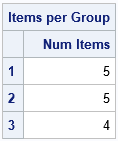The AssignItems function is a "one-liner." The interesting part of the AssignItems function is the binary expression i <= mod(B, k), which is valid even when i is a vector. In this example, the expression evaluates to the vector {1, 1, 0}, which assigns an extra item to each of the first two groups.

### Which items are assigned to each group?

A related problem is figuring out which items get sent to which groups. In the example where B=14 and k=3, I want to put items 1-5 in the first group, items 6-10 in the second group, and items 11-14 in the last group. There is a cool programming trick, called the CUSUM-LAG trick, which enables you to find these indices. The following function is copied from my article on the CUSUM-LAG trick. After you find the number of items in each group, you can use the ByGroupIndices function to find the item numbers in each group:

```/* Return kx2 matrix that contains the first and last elements for k groups that have sizes s, s,...,s[k]. The i_th row contains the first and last index for the i_th group. */ start ByGroupIndices( s ); size = colvec(s); /* make sure you have a column vector */ endIdx = cusum(size); /* locations of ending index */ beginIdx = 1 + lag(endIdx); /* begin after each ending index ... */ beginIdx = 1; /* ...and at 1 */ return ( beginIdx || endIdx ); finish;   /* apply the CUSUM-LAG trick to the item allocation problem */ GroupInfo = n || ByGroupIndices(n); print GroupInfo[r=Group c={"NumItems" "FirstItem" "LastItem"}];```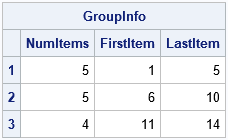The table shows the number of items allocated to each group, as well as the item indices in each group. You can use the FLOOR-MOD trick to get the number of items and the CUSUM-LAG trick to get the indices. In a vector language, you can implement the entire computation without using any loops.

The post Use the FLOOR-MOD trick to allocate items to groups appeared first on The DO Loop.As a general rule, when SAS programmers want to manipulate data row by row, they reach for the SAS DATA step. When the computation requires column statistics, the SQL procedure is also useful. When both row and column operations are required, the SAS/IML language is a powerful addition to a SAS programmer's toolbox.

I was reminded of this fact recently when a SAS programmer (possibly a student) asked how to "manually" perform the classic chi-square test for association in a two-way frequency table. The computation requires computing the means across rows and down columns, and the student was struggling with implementing the computations in the DATA step. This article illustrates how SAS/IML can simplify the rowwise and columnwise computations in the classic chi-square test.

### The chi-square test for association in PROC FREQ

In SAS, the easy way to compute the chi-square test for association is to use PROC FREQ. The following data are from several examples in the PROC FREQ documentation. The data shows the hair color and eye color of 762 European children. The call to PROC FREQ computes the chi-square test and a cross-tabulation that displays the observed value, expected values (under the hypothesis that hair color and eye color are independent), and deviations, which are the "observed minus expected" values:

```data Color; input Eyes \$ Hair \$ Count @@; label Eyes ='Eye Color' Hair ='Hair Color'; datalines; blue black 6 blue dark 51 blue fair 69 blue medium 68 blue red 28 brown black 16 brown dark 94 brown fair 90 brown medium 94 brown red 47 green dark 37 green fair 69 green medium 55 green red 38 ;   title 'Eye and Hair Color of European Children'; proc freq data=Color; tables Eyes*Hair / nopercent norow nocol deviation expected chisq; weight Count; run;```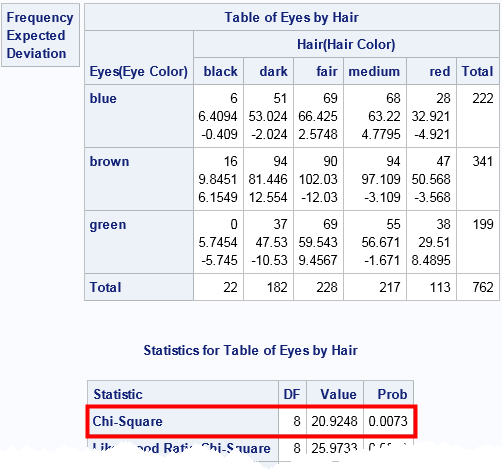In the eye-by-hair table, each cell contains three values. The first value is the observed cell count, the second value is the expected cell count (assuming independence), and the third value is their difference, which is sometimes called the "deviation." The test statistic and p-value for the chi-square test are outlined in red. The test statistic is 20.92. The probability of observing that value from a random draw of a chi-square distribution with 8 degrees of freedom is 0.0073. Because that probability is so small, we reject the null hypothesis that hair color and eye color are independent.

### Compute the chi-square test "manually" in SAS

The chi-square test on a 3 x 4 table is simple enough to compute by hand, but suppose you want to use SAS to validate or reproduce the numbers that PROC FREQ produces? This is a good programming exercise for students to make sure they understand the computations. The PROC FREQ documentation provides the formula for the test statistic by using the equation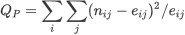where nij is the observed count in row i and column j and eij is the expected count, but there is nothing like programming a formula to ensure understanding.

The following steps indicate the (vectorized) computations that can be used to implement the chi-square test in SAS/IML.

1. Use subscript reduction operators to compute the means for each row and column, and the grand mean for all cells.
2. Use an outer product to form the table of expected values from the mean vectors.
3. Compute the test statistic by using elementwise matrix operations.
4. Use the CDF function to compute the p-value.
```proc iml; /* row means, column means, and overall mean */ cName = {"black" "dark" "fair" "medium" "red"}; rName = {"blue" "brown" "green"}; C = { 6 51 69 68 28, 16 94 90 94 47, 0 37 69 55 38}; colMarg = C[:, ]; /* mean of each column */ rowMarg = C[ ,:]; /* mean of each row */ grandMean = rowMarg[:]; /* grand mean (also C{:]) */   /* expected values under hypothesis of independence */ Expected = rowMarg*colMarg / grandMean; /* outer product */ Deviance = C - Expected; /* difference (observed-expected) for each cell */ /* chi square statistic: Sum(( Observed[i,j] - Expected[i,j])^2 / Expected[i,j] ) */ ChiSq = sum( Deviance##2 / Expected ); DF = (nrow(C)-1) * (ncol(C)-1); pvalue = 1 - cdf("ChiSq", ChiSq, DF);   print Expected[c=cName r=rName], Deviance[c=cName r=rName]; print ChiSq pvalue; quit;```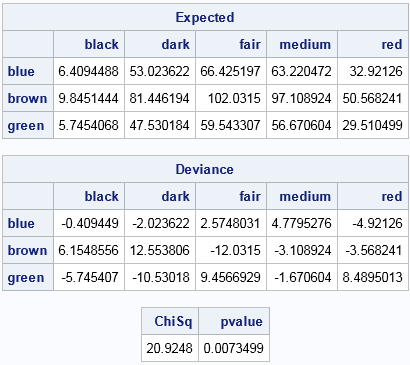Notice that the program does not contain any loops, although the formulas contain double summations over the elements of the table. This is an example of "vectorizing" the computations, which means writing the computations as vector or matrix computations rather than scalar operations in a loop.

You can see that the 'Expected' matrix matches the PROC FREQ output for the expected values for each cell. Similarly, the 'Deviance' matrix matches the PROC FREQ output for the difference between observed and expected values. The test statistic is the sum of the ratios of the squared deviances and the expected values. A call to the CDF function computes the p-value.

In summary, you can use the high-level SAS/IML language to implement basic statistical tests such as the chi-square test for association in a two-way frequency table. Such an exercise enables students to understand the details of elementary statistical tests. For programmers who know the statistical details but who are new to the SAS/IML language, this short exercise provides a way to gain proficiency with vectorized programming techniques.

The post The chi-square test: An example of working with rows and columns in SAS appeared first on The DO Loop.One way to assess the precision of a statistic (a point estimate) is to compute the standard error, which is the standard deviation of the statistic's sampling distribution. A relatively large standard error indicates that the point estimate should be viewed with skepticism, either because the sample size is small or because the data themselves have a large variance. The jackknife method is one way to estimate the standard error of a statistic.

Some simple statistics have explicit formulas for the standard error, but the formulas often assume normality of the data or a very large sample size. When your data do not satisfy the assumptions or when no formula exists, you can use resampling techniques to estimate the standard error. Bootstrap resampling is one choice, and the jackknife method is another. Unlike the bootstrap, which uses random samples, the jackknife is a deterministic method.

This article explains the jackknife method and describes how to compute jackknife estimates in SAS/IML software. This is best when the statistic that you need is also implemented in SAS/IML. If the statistic is computed by a SAS procedure, you might prefer to download and use the %JACK macro, which does not require SAS/IML.

### The jackknife method: Leave one out!

The jackknife method estimates the standard error (and bias) of statistics without making any parametric assumptions about the population that generated the data. It uses only the sample data.

The jackknife method manufactures jackknife samples from the data. A jackknife sample is a "leave-one-out" resample of the data. If there are n observations, then there are n jackknife samples, each of size n-1. If the original data are {x1, x2,..., xn}, then the i_th jackknife sample is
{x1,..., xi-1,xi+1,..., xn}
You then compute n jackknife replicates. A jackknife replicate is the statistic of interest computed on a jackknife sample. You can obtain an estimate of the standard error from the variance of the jackknife replicates. The jackknife method is summarized by the following:

1. Compute a statistic, T, on the original sample of size n.
2. For i = 1 to n, repeat the following:
• Leave out the i_th observation to form the i_th jackknife sample.
• Compute the i_th jackknife replicate statistic, Ti, by computing the statistic on the i_th jacknife sample.
3. Compute the average (mean) of the jackknife replicates: Tavg = Σi Ti / n.
4. (Optional) Estimate the bias as BiasTjack = (n-1)(Tavg - T)
5. Estimate the standard error as SEjack = sqrt( (n-1)/n (Σi Ti - Tavg)**2 )

### Data for a jackknife example

Resampling methods are not hard, but the notation in some books can be confusing. To clarify the method, let's choose a particular statistic and look at example data. The following example is from Martinez and Martinez (2001, 1st Ed, p. 241), which is also the source for this article. The data are the LSAT scores and grade-point averages (GPAs) for 15 randomly chosen students who applied to law school.

```data law; input lsat gpa @@; datalines; 653 3.12 576 3.39 635 3.30 661 3.43 605 3.13 578 3.03 572 2.88 545 2.76 651 3.36 555 3.00 580 3.07 594 2.96 666 3.44 558 2.81 575 2.74 ;```

The statistic of interest (T) will be the correlation coefficient between the LSAT and the GPA variables for the n=15 observations. The observed correlation is TData = 0.776. The standard error of T helps us understand how much T would change if we took a different random sample of 15 students. The next sections show how to implement the jackknife analysis in the SAS/IML language.

### Construct a jackknife sample in SAS

The SAS/IML matrix language is the simplest way to perform a general jackknife estimates. If X is an n x p data matrix, you can obtain the i_th jackknife sample by excluding the i_th row of X. The following two helper functions encapsulate some of the computations. The SeqExclude function returns the index vector {1, 2, ..., i-1, i+1, ..., n}. The JackSample function returns the data matrix without the i_th row:

```proc iml; /* return the vector {1,2,...,i-1, i+1,...,n}, which excludes the scalar value i */ start SeqExclude(n,i); if i=1 then return 2:n; if i=n then return 1:n-1; return (1:i-1) || (i+1:n); finish;   /* return the i_th jackknife sample for (n x p) matrix X */ start JackSamp(X,i); n = nrow(X); return X[ SeqExclude(n, i), ]; /* return data without i_th row */ finish;```

### The jackknife method for multivariate data in SAS

By using the helper functions, you can carry out each step of the jackknife method. To make the method easy to modify for other statistics, I've written a function called EvalStat which computes the correlation coefficient. This function is called on the original data and on each jackknife sample.

```/* compute the statistic in this function */ start EvalStat(X); return corr(X)[2,1]; /* <== Example: return correlation between two variables */ finish;   /* read the data into a (n x 2) data matrix */ use law; read all var {"gpa" "lsat"} into X; close;   /* 1. compute statistic on observed data */ T = EvalStat(X);   /* 2. compute same statistic on each jackknife sample */ n = nrow(X); T_LOO = j(n,1,.); /* LOO = "Leave One Out" */ do i = 1 to n; Y = JackSamp(X,i); T_LOO[i] = EvalStat(Y); end;   /* 3. compute mean of the LOO statistics */ T_Avg = mean( T_LOO );   /* 4 & 5. compute jackknife estimates of bias and standard error */ biasJack = (n-1)*(T_Avg - T); stdErrJack = sqrt( (n-1)/n * ssq(T_LOO - T_Avg) ); result = T || T_Avg || biasJack || stdErrJack; print result[c={"Estimate" "Mean Jackknife Estimate" "Bias" "Std Error"}];```The output shows that the estimate of bias for the correlation coefficient is very small. The standard error of the correlation coefficient is estimated as 0.14, which is about 18% of the estimate.

To use this code yourself, simply modify the EvalStat function. The remainder of the program does not need to change.

### The jackknife method in SAS/IML: Univariate data

When the data are univariate, you can sometimes eliminate the loop that computes jackknife samples and evaluates the jackknife replicates. If X is column vector, you can computing the (n-1) x n matrix whose i_th column represents the i_th jackknife sample. (To prevent huge matrices, this method is best for n < 20000.) Because many statistical functions in SAS/IML operate on the columns of a matrix, you can often compute the jackknife replicates in a vectorized manner.

In the following program, the JackSampMat function returns the matrix of jackknife samples for univariate data. The function calls the REMOVE function in SAS/IML, which deletes specified elements of a matrix and returns the results in a row vector. The EvalStatMat function takes the matrix of jackknife samples and returns a row vector of statistics, one for each column. In this example, the function returns the sample standard deviation.

```/* If x is univariate, you can construct a matrix where each column contains a jackknife sample. Use for univariate column vector x when n < 20000 */ start JackSampMat(x); n = nrow(x); B = j(n-1, n,0); do i = 1 to n; B[,i] = remove(x, i)`; /* transpose to column vevtor */ end; return B; finish;   /* Input: matrix where each column of X is a bootstrap sample. Return a row vector of statistics, one for each column. */ start EvalStatMat(x); return std(x); /* <== Example: return std dev of each sample */ finish;```

Let's use these functions to get a jackknife estimate of the standard error for the statistic (the standard deviation). The data (from Martinez and Martinez, p. 246) have been studied by many researchers and represent the weight gain in grams for 10 rats who were fed a low-protein diet of cereal:

```x = {58,67,74,74,80,89,95,97,98,107}; /* Weight gain (g) for 10 rats */   /* optional: visualize the matrix of jackknife samples */ *M = JackSampMat(x); *print M[c=("S1":"S10") r=("1":"9")];   /* Jackknife method for univariate data */ /* 1. compute observed statistic */ T = EvalStatMat(x); /* 2. compute same statistic on each jackknife sample */ T_LOO = EvalStatMat( JackSampMat(x) ); /* LOO = "Leave One Out" */ /* 3. compute mean of the LOO statistics */ T_Avg = mean( T_LOO` ); /* transpose T_LOO */ /* 4 & 5. compute jackknife estimates of bias and standard error */ biasJack = (n-1)*(T_Avg - T); stdErrJack = sqrt( (n-1)/n * ssq(T_LOO - T_Avg) ); result = T || T_Avg || biasJack || stdErrJack; print result[c={"Estimate" "Mean Jackknife Estimate" "Bias" "Std Error"}];```The output shows that the standard deviation of these data is about 15.7 grams. The jackknife method computes that the standard error for this statistic about 2.9 grams, which is about 18% of the estimate.

In summary, jackknife estimates are straightforward to implement in SAS/IML. This article shows a general implementation that works for all data and a specialized implementation that works for univariate data. In both cases, you can adapt the code for your use by modifying the function that computes the statistic on a data set. This approach is useful and efficient when the statistic is implemented in SAS/IML.

The post The jackknife method to estimate standard errors in SAS appeared first on The DO Loop.Many intervals in statistics have the form p ± δ, where p is a point estimate and δ is the radius (or half-width) of the interval. (For example, many two-sided confidence intervals have this form, where δ is proportional to the standard error.) Many years ago I wrote an article that mentioned that you can construct these intervals in the SAS/IML language by using a concatenation operator (|| or //). The concatenation creates a two-element vector, like this:

```proc iml; mu = 50; delta = 1.5; CI = mu - delta || mu + delta; /* horizontal concatenation ==> 1x2 vector */```

Last week it occurred to me that there is a simple trick that is even easier: use the fact that SAS/IML is a matrix-vector language to encode the "±" sign as a vector {-1, 1}. When SAS/IML sees a scalar multiplied by a vector, the result will be a vector:

```CI = mu + {-1 1}*delta; /* vector operation ==> 1x2 vector */ print CI;```You can extend this example to compute many intervals by using a single statement. For example, in elementary statistics we learn the "68-95-99.7 rule" for the normal distribution. The rule says that in a random sample drawn from a normal population, about 68% of the observations will be within 1 standard deviation of the mean, about 95% will be within 2 standard deviations, and about 99.7 % will be within 3 standard deviations of the mean. You can construct those intervals by using a "multiplier matrix" whose first row is {-1, +1}, whose second row is {-2, +2}, and whose third row is {-3, +3}. The following SAS/IML statements construct the three intervals for the 69-95-99.7 rule for a normal population with mean 50 and standard deviation 8:

```mu = 50; sigma = 8; m = {-1 1, -2 2, -3 3};   Intervals = mu + m*sigma; ApproxPct = {"68%", "95%", "99.7"}; print Intervals[rowname=ApproxPct];```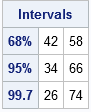Just for fun, let's simulate a large sample from the normal population and empirically confirm the 68-95-99.7 rule. You can use the RANDFUN function to generate a random sample and use the BIN function to detect which observations are in each interval:

```call randseed(12345); n = 10000; /* sample size */ x = randfun(n, "Normal", mu, sigma); /* simulate normal sample */ ObservedPct = j(3,1,0); do i = 1 to 3; b = bin(x, Intervals[i,]); /* b[i]=1 if x[i] in interval */ ObservedPct[i] = sum(b) / n; /* percentage of x in interval */ end;   results = Intervals || {0.68, 0.95, 0.997} || ObservedPct; print results[colname={"Lower" "Upper" "Predicted" "Observed"} label="Probability of Normal Variate in Intervals: X ~ N(50, 8)"];```The simulation confirms the 68-95-99.7 rule. Remember that the rule is a mnemonic device. You can compute the exact probabilities by using the CDF function. In SAS/IML, the exact computation is p = cdf("Normal", m[,2]) - cdf("Normal", m[,1]);

In summary, the SAS/IML language provides an easy syntax to construct intervals that are symmetric about a central value. You can use a vector such as {-1, 1} to construct an interval of the form p ± δ, or you can use a k x 2 matrix to construct k symmetric intervals.

The post A simple trick to construct symmetric intervals appeared first on The DO Loop.I recently needed to solve a fun programming problem. I challenge other SAS programmers to solve it, too! The problem is easy to state: Given a long sequence of digits, can you write a program to count how many times a particular subsequence occurs? For example, if I give you a sequence of 1,000 digits, can you determine whether the five-digit pattern {1 2 3 4 5} appears somewhere as a subsequence? How many times does it appear?

If the sequence is stored in a data set with one digit in each row, then SAS DATA step programmers might suspect that the LAG function will be useful for solving this problem. The LAG function enables a DATA step to examine the values of several digits simultaneously.

The SAS/IML language also has a LAG function which enables you to form a matrix of lagged values. This leads to an interesting way use vectorized computations to solve this problem. The following SAS/IML program defines a small eight-digit set of numbers in which the pattern {1 2 3} appears twice. The LAG function in SAS/IML accepts a vector of lags and creates a matrix where each column is a lagged version of the input sequence:

```/* Find a certain pattern in sequence of digits */ proc iml; Digit = {1,1,2,3,3,1,2,3}; /* digits to search */ target = {1 2 3}; /* the pattern to find */ p = ncol(target); /* length of target sequence */ D = lag(Digit, (p-1):0); /* columns shift the digits */ print D;```The output shows a three-column matrix (D) that contains the second, first, and zeroth lag (in that order) for the input sequence. Notice that if I am searching for a particular three-digit pattern, this matrix is very useful. The rows of this matrix are all three-digit patterns that appear in the original sequence. Consequently, to search for a three-digit pattern, I can use the rows of the matrix D.

To make the task easier, you can delete the first two rows, which contain missing values. You can also form a binary matrix X that has the value X[i,j]=1 when the j_th element of the pattern equals the j_th element of the i_th row, and 0 otherwise, as shown in the following:

```D = D[p:nrow(Digit),]; /* delete first p rows */ X = (D=target); /* binary matrix */ print X;```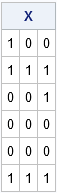Notice that in SAS/IML, the comparison operator (=) can perform a vector comparison. The binary comparison operator detects that the matrix on the left (D) and the vector on the right (target) both contain three columns. Therefore the operator creates the three-column logical matrix X, as shown. The X matrix has a wonderful property: a row of X contains all 1s if and only if the corresponding row of D matches the target pattern. So to find matches, you just need to sum the values in the rows of X. If the row sum equals the number of digits in the pattern, then that row indicates a place where the target pattern appears in the original sequence.

You can program this test in PROC IML as follows. The subscript reduction operator [,+] is shorthand for "compute the sum of each row over all columns".

```/* sum across columns. Which rows contain all 1s? */ b = (X[,+] = p); /* b[i]=1 if i_th row matches target */ NumRepl = sum(b); /* how many times does target appear? */ if NumRepl=0 then print "The target does not appear in the digits"; else print "The target appears at location " (loc(b)), /* print 1st location */ "The target appears" (NumRepl) "times.";```The program discovered that the target pattern appears in the sequence twice. The first appearance begins with the second digit in the sequence. The pattern also appears in the sequence at the sixth position, although that information is not printed.

Notice that you can solve this problem in SAS/IML without writing any loops. Instead, you can use the LAG function to convert the N-digit sequence into a matrix with N-p rows and p columns. You can then test whether the target pattern matches one of the rows.

### Your turn! Can you solve this problem?

Now that I have shown one way to solve the problem, I invite SAS programmers to write their own program to determine whether a specified pattern appears in a numeric sequence. You can use the DATA step, DS2, SQL, or any other SAS language.

Post a comment to submit your best SAS program. Extra points for programs that count all occurrences of the pattern and display the location of the first occurrence.

To help you debug your program, here is test data that we can all use. It contains 10 million random digits:

```data Have(keep=Digit); call streaminit(31488); do i = 1 to 1e7; Digit = floor(10*rand("uniform")); output; end; run;```

To help you determine if your program is correct, you can use the following results. In this sequence of digits:

• The five-digit pattern {1 2 3 4 5} occurs 101 times and the first appearance begins at row 34417
• The six-digit patter {6 5 4 3 2 1} occurs 15 times and the first appearance begins at row 120920

You can verify these facts by using PROC PRINT as follows:

```proc print data=Have(firstobs=34417 obs=34421); run; proc print data=Have(firstobs=120920 obs=120925); run;```

Happy programming!

The post Find a pattern in a sequence of digits appeared first on The DO Loop.Two of my favorite string-manipulation functions in the SAS DATA step are the COUNTW function and the SCAN function. The COUNTW function counts the number of words in a long string of text. Here "word" means a substring that is delimited by special characters, such as a space character, a period, or a comma. The SCAN function enables you to parse a long string and extract words. You can specify the delimiters yourself or use the default delimiters. Ron Cody discusses these and other string manipulation functions in his excellent 2005 tutorial, "An Introduction to SAS Character Functions."

### Using the COUNTW and SCAN functions in the DATA step

For example, the following DATA step reads in a long line of text. The COUNTW function counts how many words are in the string. A loop then iterates over the number of words and the SCAN function extracts each word into a variable:

```data parse; length word \$20; /* number of characters in the longest word */ input str \$ 1-80; delims = ' ,.!'; /* delimiters: space, comma, period, ... */ numWords = countw(str, delims); /* for each line of text, how many words? */ do i = 1 to numWords; /* split text into words */ word = scan(str, i, delims); output; end; drop str delims i; datalines; Introduction,to SAS/IML programming! Do you have... a question? ;   proc print data=parse; run;```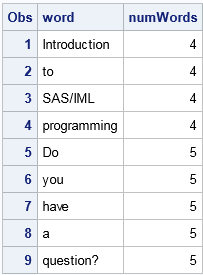Notice that the delimiters do not include the '/' or '?' characters. Therefore these characters are considered to be part of words. For example, the strings "SAS/IML" and "question?" include those non-letter characters. Notice also that consecutive delimiters are automatically excluded, such as extra spaces or the ellipses marks.

### Creating a vector of words in SAS/IML

One of the advantages of the SAS/IML matrix language is that you can call the hundreds of functions in Base SAS. When you pass in a vector of arguments to a Base SAS function, the function returns a vector that is the same size and shape as the parameter. In this way, you can vectorize the calling of Base SAS functions. In particular, you can pass in a vector of indices to the SCAN function and get back a vector of words. You do not need to write a loop to extract multiple words, as the following example demonstrates:

```proc iml; s = "Introduction,to SAS/IML... programming!"; delims = ' ,.!'; n = countw(s, delims); words = scan(s, 1:n, delims); /* pass parameter vector: create vector of words */ print words;```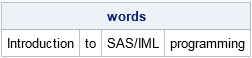In summary, Base SAS provides many useful functions such as the string manipulation functions. This article shows that when you call these functions from SAS/IML and pass in a parameter vector, you get back a vector of results.

The post Break a sentence into words in SAS appeared first on The DO Loop.